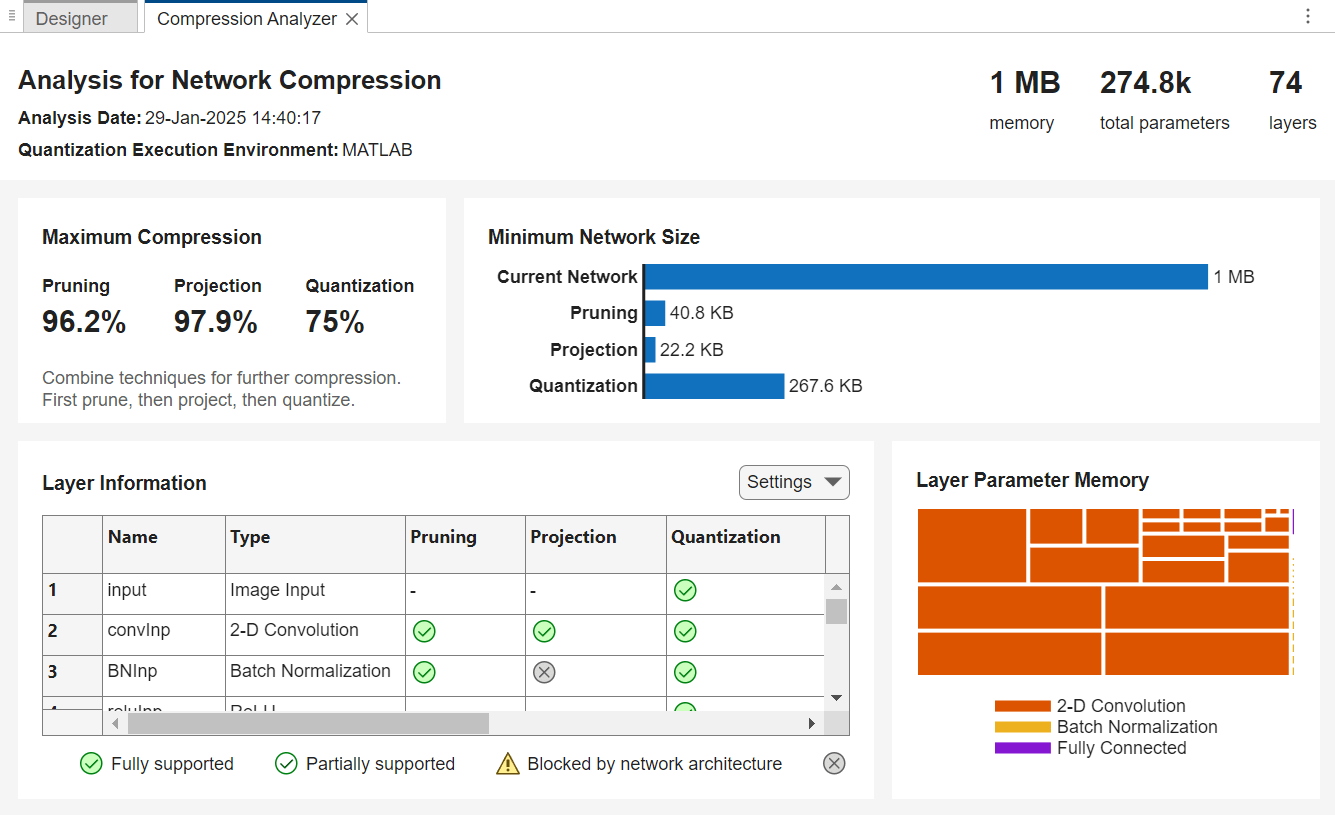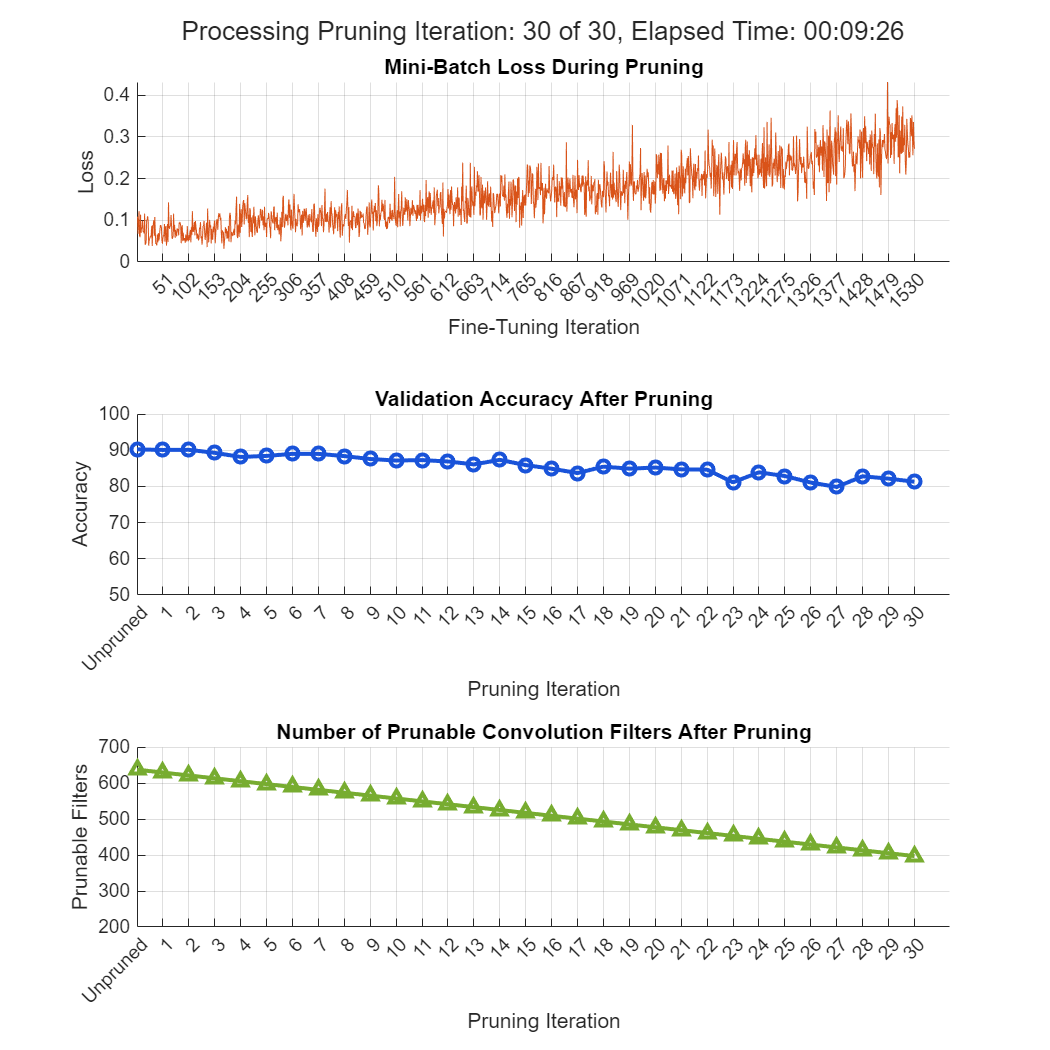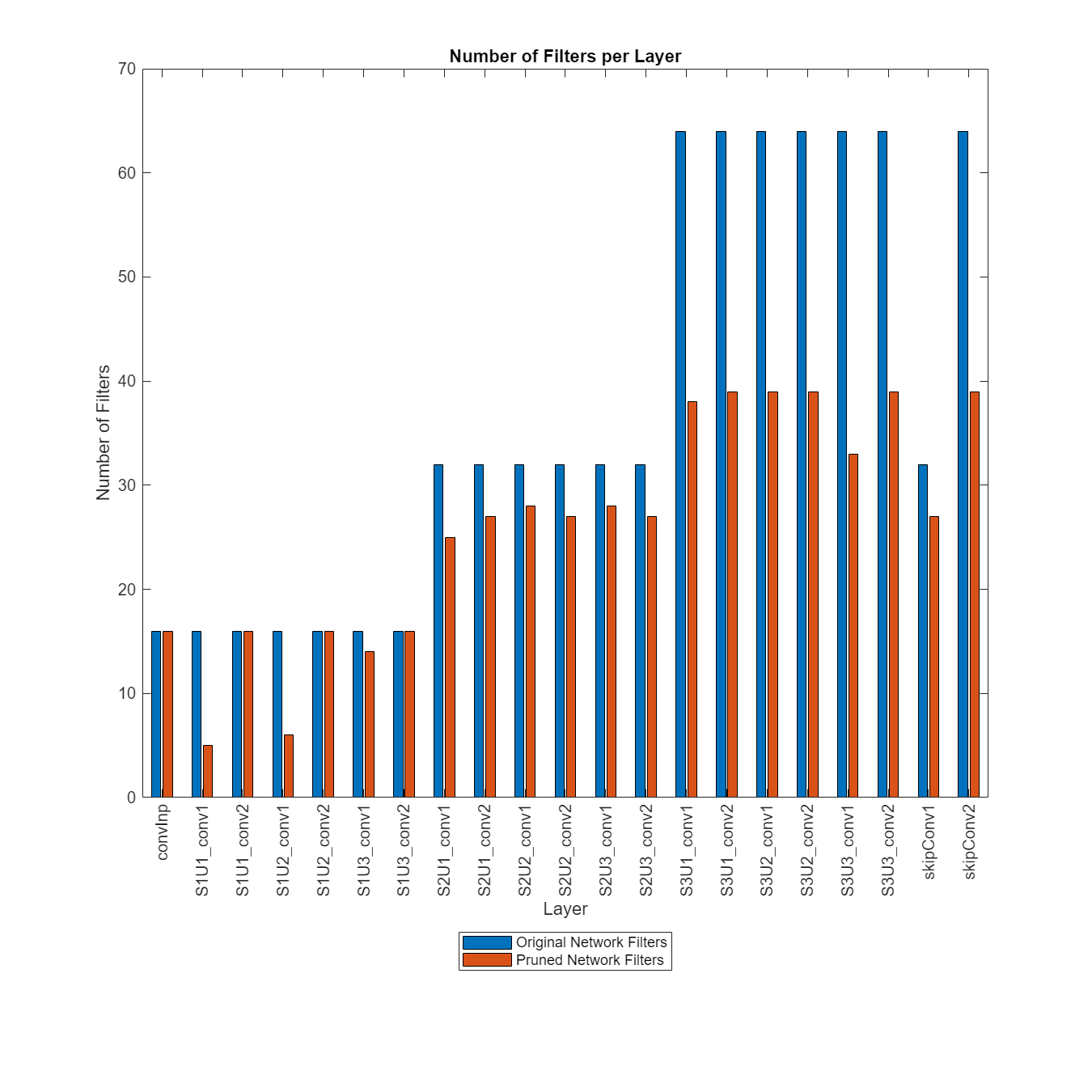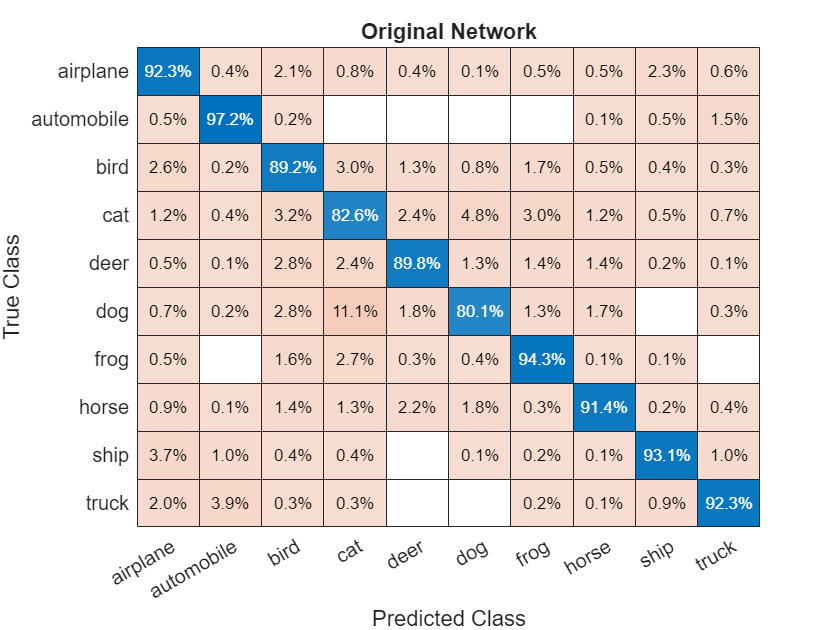# Prune Image Classification Network Using Taylor Scores

This example shows how to reduce the size of a deep neural network using Taylor pruning. By using the `taylorPrunableNetwork` function to remove convolution layer filters, you can reduce the overall network size and increase the inference speed.

Network pruning is a powerful model compression tool that helps identify redundancies that can be removed with little impact on the final network output. Pruning is particularly useful in transfer learning, where the network is often overparameterized.

This example uses a residual network trained on the CIFAR-10 data set. For more information, see Train Residual Network for Image Classification.

### Load Pretrained Network and Data

Download the CIFAR-10 data set  using the `downloadCIFARData` function, attached to this example as a supporting file. To access this file, open the example as a live script. The data set contains 60,000 images. Each image is 32-by-32 in size and has three color channels (RGB). The size of the data set is 175 MB. Depending on your internet connection, the download process can take time.

```datadir = tempdir; downloadCIFARData(datadir);```

Load the trained network for pruning.

`load("CIFARNet-20-16.mat","trainedNet");`

Load the CIFAR-10 training and test images as 4-D arrays. The training set contains 50,000 images and the test set contains 10,000 images. Convert the images to an `augmentedImageDatastore` for training and validation.

```[XTrain,TTrain,XTest,TTest] = loadCIFARData(datadir); inputSize = trainedNet.Layers(1).InputSize; augimdsTrain = augmentedImageDatastore(inputSize,XTrain,TTrain); augimdsTest = augmentedImageDatastore(inputSize,XTest,TTest); classes = categories(TTest);```

Calculate the accuracy of the trained network on the test data.

```YTest = classify(trainedNet,augimdsTest); accuracyOfTrainedNet = mean(YTest == TTest)*100```
```accuracyOfTrainedNet = 90.2400 ```

To implement a custom pruning loop, convert the network to a `dlnetwork` object.

```layerG = layerGraph(trainedNet); layerG = removeLayers(layerG,layerG.OutputNames); net = dlnetwork(layerG);```

Analyze the network. As the network diagram shows, conversion removes the classification layer of the network. The deep network analyzer shows the total number of learnable parameters in the network.

`analyzeNetwork(net)`

### Prune Network

Prune the network using the t`aylorPrunableNetwork` function. The network computes an importance score for each convolution filter in the network based on Taylor expansion . Pruning is iterative: each time the loop runs, until a stopping criterion is met, the function removes a small number of the least important convolution filters and updates the network architecture.

#### Specify Pruning and Fine-Tuning Options

Set the pruning options.

• `maxPruningIterations` sets the maximum number of iterations to be used for pruning process

• `maxToPrune` is set as the maximum number of filters to be pruned in each iteration of the pruning cycle

```maxPruningIterations = 30; maxToPrune = 8;```

Set the fine-tuning options.

```learnRate = 1e-2; momentum = 0.9; miniBatchSize = 256; numMinibatchUpdates = 50; validationFrequency = 1;```

#### Prune Network using Custom Pruning Loop

Create a Taylor prunable network from the original network.

```prunableNet = taylorPrunableNetwork(net); maxPrunableFilters = prunableNet.NumPrunables;```

Create a `minibatchqueue` object that processes and manages mini-batches of images during training. For each mini-batch:

• Use the custom mini-batch preprocessing function `preprocessMiniBatch` (defined at the end of this example) to convert the labels to one-hot encoded variables.

• Format the image data with the dimension labels `'SSCB'` (spatial, spatial, channel, batch). By default, the `minibatchqueue` object converts the data to `dlarray` objects with underlying type `single`. Do not add a format to the class labels.

• Train on a GPU if one is available. By default, the `minibatchqueue` object converts each output to a `gpuArray` if a GPU is available. Using a GPU requires Parallel Computing Toolbox™ and a supported GPU device. For information on supported devices, see GPU Computing Requirements (Parallel Computing Toolbox).

```mbqTrain = minibatchqueue(augimdsTrain, ... MiniBatchSize = miniBatchSize, ... MiniBatchFcn = @preprocessMiniBatchTraining, ... OutputAsDlarray = [1 1], ... OutputEnvironment = ["auto","auto"], ... PartialMiniBatch = "return", ... MiniBatchFormat = ["SSCB",""]); mbqTest = minibatchqueue(augimdsTest,... MiniBatchSize = miniBatchSize,... MiniBatchFcn = @preprocessMiniBatchTraining, ... OutputAsDlarray = [1 1], ... OutputEnvironment = ["auto","auto"], ... PartialMiniBatch = "return", ... MiniBatchFormat = ["SSCB",""]);```

Initialize the training progress plots.

```figure("Position",[10,10,700,700]) tl = tiledlayout(3,1); lossAx = nexttile; lineLossFinetune = animatedline(Color=[0.85 0.325 0.098]); ylim([0 inf]) xlabel("Fine-Tuning Iteration") ylabel("Loss") grid on title("Mini-Batch Loss During Pruning") xTickPos = []; accuracyAx = nexttile; lineAccuracyPruning = animatedline(Color=[0.098 0.325 0.85],LineWidth=2,Marker="o"); ylim([50 100]) xlabel("Pruning Iteration") ylabel("Accuracy") grid on addpoints(lineAccuracyPruning,0,accuracyOfTrainedNet) title("Validation Accuracy After Pruning") numPrunablesAx = nexttile; lineNumPrunables = animatedline(Color=[0.4660 0.6740 0.1880],LineWidth=2,Marker="^"); ylim([200 700]) xlabel("Pruning Iteration") ylabel("Prunable Filters") grid on addpoints(lineNumPrunables,0,double(maxPrunableFilters)) title("Number of Prunable Convolution Filters After Pruning")```

Prune the network by repeatedly fine-tuning the network and removing the low scoring filters.

For each pruning iteration. The following steps are used:

• Fine-tune network and accumulate Taylor scores for convolution filters for `numMinibatchUpdates`

• Prune the network using the `updatePrunables` function to remove `maxToPrune` number of convolution filters

• Compute validation accuracy

To fine tune the network, loop over the mini-batches of the training data. For each mini-batch in the fine-tuning iteration the following steps are used:

• Evaluate the pruning loss, gradients of the pruning activations, pruning activations, model gradients and the state using the `dlfeval` and `modelLossPruning` functions.

• Update the network state.

• Update the network parameters using the `sgdmupdate` function.

• Update the Taylor scores of the prunable network using the `updateScore` function.

• Display the training progress.

```start = tic; iteration = 0; for pruningIteration = 1:maxPruningIterations % Shuffle data. shuffle(mbqTrain); % Reset the velocity parameter for the SGDM solver in every pruning % iteration. velocity = []; % Loop over mini-batches. fineTuningIteration = 0; while hasdata(mbqTrain) iteration = iteration + 1; fineTuningIteration = fineTuningIteration + 1; % Read mini-batch of data. [X, T] = next(mbqTrain); % Evaluate the pruning activations, gradients of the pruning % activations, model gradients, state, and loss using the dlfeval and % modelLossPruning functions. [loss,pruningActivations, pruningGradients, netGradients, state] = ... dlfeval(@modelLossPruning, prunableNet, X, T); % Update the network state. prunableNet.State = state; % Update the network parameters using the SGDM optimizer. [prunableNet, velocity] = sgdmupdate(prunableNet, netGradients, velocity, learnRate, momentum); % Compute first-order Taylor scores and accumulate the score across % previous mini-batches of data. prunableNet = updateScore(prunableNet, pruningActivations, pruningGradients); % Display the training progress. D = duration(0,0,toc(start),Format="hh:mm:ss"); addpoints(lineLossFinetune, iteration, double(loss)) title(tl,"Processing Pruning Iteration: " + pruningIteration + " of " + maxPruningIterations + ... ", Elapsed Time: " + string(D)) % Synchronize the x-axis of the accuracy and numPrunables plots with the loss plot. xlim(accuracyAx,lossAx.XLim) xlim(numPrunablesAx,lossAx.XLim) drawnow % Stop the fine-tuning loop when numMinibatchUpdates is reached. if (fineTuningIteration > numMinibatchUpdates) break end end % Prune filters based on previously computed Taylor scores. prunableNet = updatePrunables(prunableNet, MaxToPrune = maxToPrune); % Show results on the validation data set in a subset of pruning iterations. isLastPruningIteration = pruningIteration == maxPruningIterations; if (mod(pruningIteration, validationFrequency) == 0 || isLastPruningIteration) accuracy = modelAccuracy(prunableNet, mbqTest, classes, augimdsTest.NumObservations); addpoints(lineAccuracyPruning, iteration, accuracy) addpoints(lineNumPrunables,iteration,double(prunableNet.NumPrunables)) end % Set x-axis tick values at the end of each pruning iteration. xTickPos = [xTickPos, iteration]; %#ok<AGROW> xticks(lossAx,xTickPos) xticks(accuracyAx,[0,xTickPos]) xticks(numPrunablesAx,[0,xTickPos]) xticklabels(accuracyAx,["Unpruned",string(1:pruningIteration)]) xticklabels(numPrunablesAx,["Unpruned",string(1:pruningIteration)]) drawnow end```In contrast to typical training where the loss decreases with each iteration, pruning may increase the loss and reduce the validation accuracy due to the change of network structure when convolution filters are pruned. To further improve the accuracy of the network, you can retrain the network.

Once pruning is complete, convert the `taylorPrunableNetwork` back to a `dlnetwork` for retraining.

`prunedNet = dlnetwork(prunableNet);`

### Retrain Network After Pruning

Retrain the network after pruning to regain any loss in accuracy. To retrain the network using the `trainNetwork` function, convert the pruned network from a dlnetwork to a `layerGraph`. You can also use a custom training loop to train the network. For more information, see Train Network using Custom Training Loop.

• Extract the `layerGraph` from the `dlnetwork`.

• Add the removed classification layer from the original network to the `layerGraph` of the pruned network.

• Train the `layerGraph` network.

```prunedLayerGraph = layerGraph(prunedNet); outputLayerName = string(trainedNet.OutputNames{1}); outputLayerIdx = {trainedNet.Layers.Name} == outputLayerName; prunedLayerGraph = addLayers(prunedLayerGraph,trainedNet.Layers(outputLayerIdx)); prunedLayerGraph = connectLayers(prunedLayerGraph,prunedNet.OutputNames{1},outputLayerName);```

Set the options to the default settings for stochastic gradient descent with momentum. Set the maximum number of retraining epochs at 10 and start the training with an initial learning rate of 0.01.

```options = trainingOptions("sgdm", ... MaxEpochs = 10, ... MiniBatchSize = 256, ... InitialLearnRate = 1e-2, ... LearnRateSchedule = "piecewise", ... LearnRateDropFactor = 0.1, ... LearnRateDropPeriod = 2, ... L2Regularization = 0.02, ... ValidationData = augimdsTest, ... ValidationFrequency = 200, ... Verbose = false, ... Shuffle = "every-epoch", ... Plots = "training-progress");```

Train the network.

`prunedDAGNet = trainNetwork(augimdsTrain,prunedLayerGraph,options);`### Compare Original Network and Pruned Network

Determine the impact of pruning on each layer.

```[originalNetFilters,layerNames] = numConvLayerFilters(trainedNet); prunedNetFilters = numConvLayerFilters(prunedDAGNet);```

Visualize the number of filters in the original network and in the pruned network.

```figure("Position",[10,10,900,900]) bar([originalNetFilters,prunedNetFilters]) xlabel("Layer") ylabel("Number of Filters") title("Number of Filters Per Layer") xticks(1:(numel(layerNames))) xticklabels(layerNames) xtickangle(90) ax = gca; ax.TickLabelInterpreter = "none"; legend("Original Network Filters","Pruned Network Filters","Location","southoutside")```Large differences between the number of filters of the two networks indicate where many of the less important filters have been pruned.

Next, compare the accuracy of the original network and the pruned network.

```YPredOriginal = classify(trainedNet,augimdsTest); accuOriginal = mean(YPredOriginal == TTest)```
```accuOriginal = 0.9024 ```
```YPredPruned = classify(prunedDAGNet,augimdsTest); accuPruned = mean(YPredPruned == TTest)```
```accuPruned = 0.8736 ```

Pruning can unequally affect the classification of different classes and introduce bias into the model, which might not be apparent from the accuracy value. To assess the impact of pruning at a class level, use a confusion matrix chart.

```figure confusionchart(TTest,YPredOriginal,Normalization = "row-normalized"); title("Original Network")``````figure confusionchart(TTest,YPredPruned,Normalization = "row-normalized"); title("Pruned Network")```Next, estimate the model parameters for the original network and the pruned network to understand the impact of pruning on the overall network learnables and size.

`analyzeNetworkMetrics(trainedNet,prunedDAGNet,accuOriginal,accuPruned)`
```ans=3×3 table Network Learnables Approx. Network Memory (MB) Accuracy __________________ ___________________________ ________ Original Network 2.7169e+05 1.0364 0.9024 Pruned Network 1.2128e+05 0.46266 0.8736 Percentage Change -55.36 -55.36 -3.1915 ```

This table compares the size and classification accuracy of the original and the pruned network. A decrease in network memory and similar accuracy values indicate a good pruning operation. For an example showing how to further reduce the size of the network for deployment using quantization, see Quantize Residual Network Trained for Image Classification and Generate CUDA Code.

### Helper Functions

#### Evaluate Model Accuracy

The `modelAccuracy` function takes as input the network(`dlnetwork`), `minibatchque` object, the classes and the number of observations and returns the accuracy.

```function accuracy = modelAccuracy(net, mbq, classes, numObservations) % This function computes the model accuracy of a net(dlnetwork) on the minibatchque 'mbq'. totalCorrect = 0; classes = int32(categorical(classes)); reset(mbq); while hasdata(mbq) [dlX, Y] = next(mbq); dlYPred = extractdata(predict(net, dlX)); YPred = onehotdecode(dlYPred,classes,1)'; YReal = onehotdecode(Y,classes,1)'; miniBatchCorrect = nnz(YPred == YReal); totalCorrect = totalCorrect + miniBatchCorrect; end accuracy = totalCorrect / numObservations * 100; end```

#### Model Gradients Function for Fine-Tuning and Pruning

The `modelLossPruning` function takes as input a `deep.prune.TaylorPrunableNetwork` object `prunableNet`, a mini-batch of input data `X` with corresponding labels T and returns the loss, gradients of the loss with respect to the pruning activations, pruning activations, gradients of the loss with respect to the learnable parameters in `prunableNet `and the network state. To compute the gradients automatically, use the `dlgradient` function.

```function [loss,pruningGradient,pruningActivations,netGradients,state] = modelLossPruning(prunableNet, X, T) [dlYPred,state,pruningActivations] = forward(prunableNet,X); loss = crossentropy(dlYPred,T); [pruningGradient,netGradients] = dlgradient(loss,pruningActivations,prunableNet.Learnables); end```

#### Mini-Batch Preprocessing Function

The `preprocessMiniBatchTraining` function preprocesses a mini-batch of predictors and labels for loss computation during training.

```function [X,T] = preprocessMiniBatchTraining(XCell,TCell) % Concatenate. X = cat(4,XCell{1:end}); % Extract label data from cell and concatenate. T = cat(2,TCell{1:end}); % One-hot encode labels. T = onehotencode(T,1); end```

#### Evaluate Number of Filters in Convolution Layers

The `numConvLayerFilters` function returns the number of filters in each convolution layer.

```function [nFilters, convNames] = numConvLayerFilters(net) numLayers = numel(net.Layers); convNames = []; nFilters = []; % Check for convolution layers and extract the number of filters. for cnt = 1:numLayers if isa(net.Layers(cnt),"nnet.cnn.layer.Convolution2DLayer") sizeW = size(net.Layers(cnt).Weights); nFilters = [nFilters; sizeW(end)]; convNames = [convNames; string(net.Layers(cnt).Name)]; end end end```

#### Evaluate the network statistics of original network and pruned network

The `analyzeNetworkMetrics` function takes input as the original network, pruned network, accuracy of original network and the accuracy of the pruned network and returns the different statistics like network learnables, network memory and the accuracy on the test data in form of a table.

```function [statistics] = analyzeNetworkMetrics(originalNet,prunedNet,accuracyOriginal,accuracyPruned) originalNetMetrics = estimateNetworkMetrics(originalNet); prunedNetMetrics = estimateNetworkMetrics(prunedNet); % Accuracy of original network and pruned network perChangeAccu = 100*(accuracyPruned - accuracyOriginal)/accuracyOriginal; AccuracyForNetworks = [accuracyOriginal;accuracyPruned;perChangeAccu]; % Total learnables in both networks originalNetLearnables = sum(originalNetMetrics(1:end,"NumberOfLearnables").NumberOfLearnables); prunedNetLearnables = sum(prunedNetMetrics(1:end,"NumberOfLearnables").NumberOfLearnables); percentageChangeLearnables = 100*(prunedNetLearnables - originalNetLearnables)/originalNetLearnables; LearnablesForNetwork = [originalNetLearnables;prunedNetLearnables;percentageChangeLearnables]; % Approximate parameter memory approxOriginalMemory = sum(originalNetMetrics(1:end,"ParameterMemory (MB)").("ParameterMemory (MB)")); approxPrunedMemory = sum(prunedNetMetrics(1:end,"ParameterMemory (MB)").("ParameterMemory (MB)")); percentageChangeMemory = 100*(approxPrunedMemory - approxOriginalMemory)/approxOriginalMemory; NetworkMemory = [ approxOriginalMemory; approxPrunedMemory; percentageChangeMemory]; % Create the summary table statistics = table(LearnablesForNetwork,NetworkMemory,AccuracyForNetworks, ... 'VariableNames',["Network Learnables","Approx. Network Memory (MB)","Accuracy"], ... 'RowNames',{'Original Network','Pruned Network','Percentage Change'}); end```

References

 Krizhevsky, Alex. 2009. "Learning Multiple Layers of Features from Tiny Images." https://www.cs.toronto.edu/~kriz/learning-features-2009-TR.pdf.

 Molchanov, Pavlo, Stephen Tyree, Tero Karras, Timo Aila, and Jan Kautz. “Pruning Convolutional Neural Networks for Resource Efficient Inference.” Preprint, submitted June 8, 2017. https://arxiv.org/abs/1611.06440.

 Molchanov, Pavlo, Arun Mallya, Stephen Tyree, Iuri Frosio, and Jan Kautz. “Importance Estimation for Neural Network Pruning.” In 2019 IEEE/CVF Conference on Computer Vision and Pattern Recognition (CVPR), 11256–64. Long Beach, CA, USA: IEEE, 2019. https://doi.org/10.1109/CVPR.2019.01152.